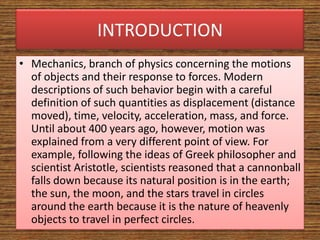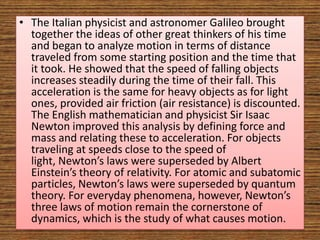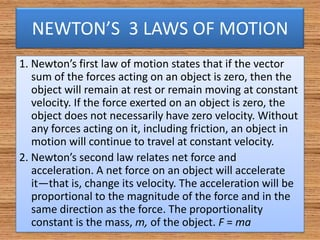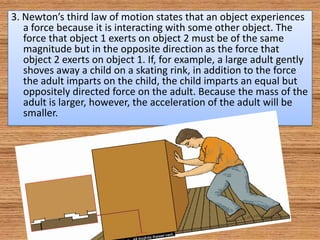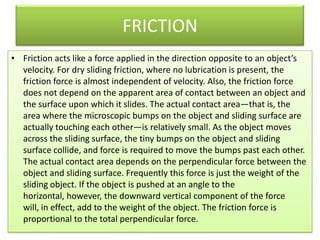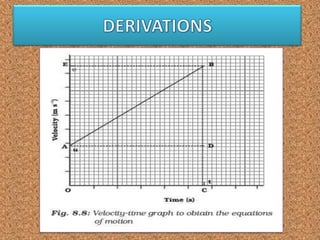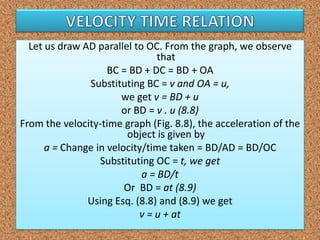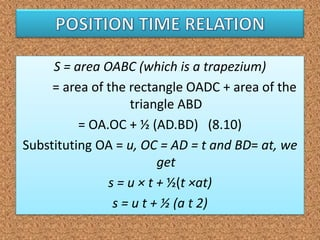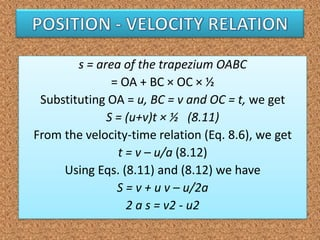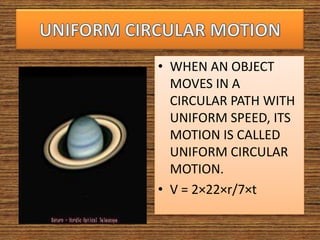1 de 11

### M O T I O N ( P H Y S I C S I T E R M )

1. MOTIO N
2. INTRODUCTION • Mechanics, branch of physics concerning the motions of objects and their response to forces. Modern descriptions of such behavior begin with a careful definition of such quantities as displacement (distance moved), time, velocity, acceleration, mass, and force. Until about 400 years ago, however, motion was explained from a very different point of view. For example, following the ideas of Greek philosopher and scientist Aristotle, scientists reasoned that a cannonball falls down because its natural position is in the earth; the sun, the moon, and the stars travel in circles around the earth because it is the nature of heavenly objects to travel in perfect circles.
3. • The Italian physicist and astronomer Galileo brought together the ideas of other great thinkers of his time and began to analyze motion in terms of distance traveled from some starting position and the time that it took. He showed that the speed of falling objects increases steadily during the time of their fall. This acceleration is the same for heavy objects as for light ones, provided air friction (air resistance) is discounted. The English mathematician and physicist Sir Isaac Newton improved this analysis by defining force and mass and relating these to acceleration. For objects traveling at speeds close to the speed of light, Newton’s laws were superseded by Albert Einstein’s theory of relativity. For atomic and subatomic particles, Newton’s laws were superseded by quantum theory. For everyday phenomena, however, Newton’s three laws of motion remain the cornerstone of dynamics, which is the study of what causes motion.
4. NEWTON’S 3 LAWS OF MOTION 1. Newton’s first law of motion states that if the vector sum of the forces acting on an object is zero, then the object will remain at rest or remain moving at constant velocity. If the force exerted on an object is zero, the object does not necessarily have zero velocity. Without any forces acting on it, including friction, an object in motion will continue to travel at constant velocity. 2. Newton’s second law relates net force and acceleration. A net force on an object will accelerate it—that is, change its velocity. The acceleration will be proportional to the magnitude of the force and in the same direction as the force. The proportionality constant is the mass, m, of the object. F = ma
5. 3. Newton’s third law of motion states that an object experiences a force because it is interacting with some other object. The force that object 1 exerts on object 2 must be of the same magnitude but in the opposite direction as the force that object 2 exerts on object 1. If, for example, a large adult gently shoves away a child on a skating rink, in addition to the force the adult imparts on the child, the child imparts an equal but oppositely directed force on the adult. Because the mass of the adult is larger, however, the acceleration of the adult will be smaller.
6. FRICTION • Friction acts like a force applied in the direction opposite to an object’s velocity. For dry sliding friction, where no lubrication is present, the friction force is almost independent of velocity. Also, the friction force does not depend on the apparent area of contact between an object and the surface upon which it slides. The actual contact area—that is, the area where the microscopic bumps on the object and sliding surface are actually touching each other—is relatively small. As the object moves across the sliding surface, the tiny bumps on the object and sliding surface collide, and force is required to move the bumps past each other. The actual contact area depends on the perpendicular force between the object and sliding surface. Frequently this force is just the weight of the sliding object. If the object is pushed at an angle to the horizontal, however, the downward vertical component of the force will, in effect, add to the weight of the object. The friction force is proportional to the total perpendicular force.
7. Let us draw AD parallel to OC. From the graph, we observe that BC = BD + DC = BD + OA Substituting BC = v and OA = u, we get v = BD + u or BD = v . u (8.8) From the velocity-time graph (Fig. 8.8), the acceleration of the object is given by a = Change in velocity/time taken = BD/AD = BD/OC Substituting OC = t, we get a = BD/t Or BD = at (8.9) Using Esq. (8.8) and (8.9) we get v = u + at
8. S = area OABC (which is a trapezium) = area of the rectangle OADC + area of the triangle ABD = OA.OC + ½ (AD.BD) (8.10) Substituting OA = u, OC = AD = t and BD= at, we get s = u × t + ½(t ×at) s = u t + ½ (a t 2)
9. s = area of the trapezium OABC = OA + BC × OC × ½ Substituting OA = u, BC = v and OC = t, we get S = (u+v)t × ½ (8.11) From the velocity-time relation (Eq. 8.6), we get t = v – u/a (8.12) Using Eqs. (8.11) and (8.12) we have S = v + u v – u/2a 2 a s = v2 - u2
10. • WHEN AN OBJECT MOVES IN A CIRCULAR PATH WITH UNIFORM SPEED, ITS MOTION IS CALLED UNIFORM CIRCULAR MOTION. • V = 2×22×r/7×t